# 6 Limits, l’Hopital’s rule, Maximum, Minimum and Calculus of Variations#

## 6 l’ Hopital’s rule#

In many calculations limits are encountered. For example, in the theory of diffraction the function $$\displaystyle \frac{\sin(x)}{x}$$ is met which, when $$x \to 0$$, has the form $$0/0$$ and at first sight this ratio seem to be indeterminate. There are other forms similar to this such as $$\infty/\infty,\, \infty/0,\, 0\times \infty,\, 0\times 0,\, \infty-\infty$$ and l’Hopital’s rule is a method, sometimes used with a little additional ingenuity, of determining these limits. This topic is discussed here, not only because it requires differentiation, but also because it will be needed in the next section. It seems that Johann Bernoulli first worked this out, but it is named after l’Hopital who was one of his pupils.

The method is

(a) Rearrange the limit required, if necessary, so that it becomes a ratio. This may require some cunning.

(b) Differentiate top and bottom separately with respect to the limit variable.

(c) Substitute in the limit and check if the ratio is still indeterminate. If it is return to (b) if not the answer has been found.

An example should make this clearer; the ratio $$\displaystyle \frac{\sin(x)}{x}$$ appears to be $$0/0$$ as $$x \to 0$$ but it has a finite value which is determined using l’Hopital’s Rule:

$\displaystyle \lim_{x\to o} \frac{\sin(x)}{x} \to \frac{\frac{d}{dx}\sin(x)}{\frac{d}{dx}x}=\frac{\cos(x)}{1}=1$

and the limiting value of $$x = 0$$ is only applied in the last step. As a check, one way to determine any limits close to zero is to plot the function. You will see that $$\displaystyle \lim_{x\to 0}\frac{\sin(x)}{x}$$ does indeed have a value of 1 at $$x = 0$$. See question 47 for other examples.

Expressions such as $$\displaystyle \frac{e^x-1}{x^2}$$ often need to be evaluated when $$x \to 0$$ or $$x \to \infty$$. In statistical mechanics, for example, $$x$$ may be $$-E/k_BT$$, a ratio of energies where $$k_B$$ is the Boltzmann constant and $$T$$ temperature. When $$x \to 0$$, which corresponds to high temperatures, the function $$\displaystyle(e^x - 1)/x^2$$ appears to have the indeterminate form $$0/0$$, but the limit by l’Hopital’s is

$\displaystyle \lim_{x\to 0}\frac{e^x -1}{x^2}\to \frac{e^x}{2x}\to \frac{e^x}{2}=\frac{1}{2} \text{ this is wrong!}$

where differentiation was performed twice over. However, this is wrong: no check for an indeterminate ratio was made after the first differentiation and doing this gives,

$\displaystyle \lim_{x\to 0}\frac{e^x -1}{x^2}\to \frac{e^x}{2x}= \frac{1}{0}=\infty$

The other limiting case $$x \to \infty$$ is also infinity: note that after the first differentiation checking the ratio gives $$\displaystyle \frac{e^x}{2x} = \frac{\infty}{\infty}$$ so indeterminate and so continue to differentiate again

$\displaystyle \lim_{x\to \infty}\frac{e^x -1}{x^2}\to \frac{e^x}{2x}\to \frac{e^x}{2}=\infty$

and this result is because $$e^x$$ increases faster with $$x$$ than $$x^2$$ does. You could plot a graph to see that this is true.

The limit $$\displaystyle \lim_{x\to\infty} \frac{e^{-x}-1}{x^2-3}$$ can perhaps be appreciated by looking at the ratio and noticing that when $$x$$ is large $$e^{-x}$$ becomes small but $$x^2$$ large so the limit is expected to be zero. Checking this properly gives the same result as intuition:

$\displaystyle \lim_{x\to \infty}\frac{e^{-x} -1}{x^2-3}\to \frac{-e^{-x}}{2x}\to \frac{e^{-x}}{2}=0$

When the limit is a product this must be rearranged first, for instance $$\displaystyle \lim_{x\to 0} x \ln(x)$$ should be rearranged to

$\displaystyle \lim_{x\to 0} \frac{ \ln(x) }{ 1/x } \to \frac{1}{ x(-x^{-2})}=-x \to 0$

Fractions are treated similarly; the following fraction is nominally undefined as $$\infty -\infty$$ but is rearranged into a ratio to become undefined as $$0/0$$;

$\displaystyle \lim_{x\to 0} \left(\frac{1}{x}-\frac{1}{\sin(x)}\right)= \lim_{x\to 0} \frac{\sin(x)-x}{x\sin(x)}\to \frac{\cos(x)-1}{x\cos(x)+\sin(x)} \to \frac{-\sin(x)}{-x\sin(x)+2\cos(x)} \to 0$

In the last step substituting for $$x=0$$ gives the ratio as $$0/1$$ so the limit is zero.

### Chemical equilibria#

In a second order reaction of the form

$\displaystyle A+B \overset{k_1}{\underset{k_{-1}} \rightleftharpoons } C+D$

the rate of change of $$C$$ is

$\displaystyle \frac{dC}{dt}=k_1AB-k_{-1}CD$

where $$C\equiv [C]$$ etc. for clarity. This can be re-written using $$A_0-A=B_0-B=C_0+C=D_0+D$$, where the subscript $$0$$ indicates the initial amount, and if initially only $$A$$ and $$B$$ are present,

$\displaystyle \frac{dC}{dt} = k_1(A_0-C)(B_0-C) - k_{-1}C^2$

which can be integrated but we are interested in the equilibrium when the rate of change is zero. Letting $$C_e$$ be the equilibrium amount gives

$\displaystyle K_e = \frac{k_1}{k_{-1}}=\frac{C_e^2}{(A_0-C_e)(B_0-C_e)}$

and when $$A_0 = B_0$$ this simplifies to

$\displaystyle K_e = \frac{k_1}{k_{-1}}=\frac{C_e^2}{(A_0-C_e)^2}$

which has the solution

$\displaystyle C_e=\frac{A_0(K_e-\sqrt{K_e})}{K_e-1}$

To find the concentration when $$K_e=1$$ the fraction seems to be $$0/\infty$$. Using L’Hopital’s rule and differentiating with $$K_e$$ gives

$\displaystyle \frac{A_0(K_e-\sqrt{K_e})}{K_e-1}\to \frac{A_0(1-\frac{1}{2}K_e^{-1/2})}{1}=\frac{A_0}{2}$

which makes sense as there are equal moles initially of $$A$$ and $$B$$ so in total $$2A_0$$ and equal concentrations for each species but only in this particular case.

### Diffraction intensity#

The intensity of line of $$N$$ emitters, such as point sources that are also coherent as occurs in the theory of interference and diffraction, is given by

$\displaystyle I=I_0\frac{\sin^2(N\delta/2)}{\sin^2(\delta/2)}$

where $$I_0$$ is the initial intensity of each emitter and $$I$$ the intensity when the phase difference between emitters is $$\delta$$. As each emitter radiates over all $$4\pi$$ angles only at certain values of these angles do the waves add up, i.e. they are in phase and so form a maxima and the equation describes how the intensity changes with phase. See Chapters 9 & 10 of ‘Optics’ by Hecht & Zajac (1982).

When $$N=1, I=I_0$$ and when $$N=2,I=4I_0\cos^2(\delta/2)$$, but what is the maximum intensity for any $$N$$? To find this we can use l’Hopital’s rule with $$\delta=2m\pi,\;m=0,\pm 1,\pm 2\cdots$$ meaning that the phase difference must be equivalent to a whole number of wavelengths for the intensity to be at a maximum. Simplifying gives

$\displaystyle \frac{\sin^2(N\delta/2)}{\sin^2(\delta/2)} = \left(\frac{\sin(N\delta/2)}{\sin(\delta/2)}\right)^2$

thus we need only

$\displaystyle \lim_{\delta\to 0}\frac{\sin(N\delta/2)}{\sin(\delta/2)}\to\frac{N\cos(N\delta/2)/2}{\cos(\delta/2)/2}=N$

making the maximum intensity $$I=N^2I_0$$ when $$\delta=2\pi m$$ which makes the cosines $$1$$. See fig 15 in chapter 9, ‘Fourier Transforms’ for a figure of the sinc function where $$\mathrm{sinc}(ax)=\sin(ax)/\sin(x)$$.

## 6.1 Beware of ‘False fractions’#

Sometimes a limit is required but the expression is not really a fraction, for example $$\displaystyle \lim_{x\to 1} \frac{x^4-1}{x-1}$$ which looks like a fraction but is simplified to $$\displaystyle \lim_{x\to 1} \frac{(x-1)(x^3+x^2+x+1)}{(x-1)}$$ and because the value $$x = 1$$ is never reached ( we are seeking the limit to not the value at $$x = 1$$) the $$x-1$$ terms can be cancelled out leaving $$\lim_{x\to 1} (x^3+x^2+x+1)=4$$

## 7 Extrema: maxima, minima and inflection points#

One very useful property of derivatives is that they allow us to find the maxima and minima of functions; these are also called stationary points of the function. The extrema might be the maximum or minimum but can also be the limit where the function goes to $$\pm \infty$$.

The maximum of a curve is a point in whose locality all surrounding points have smaller $$y$$ values. The minimum is defined similarly but points adjacent to it have larger y values and in both case the gradient is exactly zero. In Fig. 12 it is clear that the gradient is zero at the maximum and again at the minimum of the curve. The graph shows the first $$f'$$ and second $$f''$$ derivatives. An inflexion point can occur when the gradient is zero but $$y$$ is smaller on one side of the point than it is on the other. An inflexion can also occur when the gradient is not zero in which case the curvature of the line changes from concave to convex or vice versa.

The first derivative is zero at $$x$$ = 4/3 and 3, which are the maximum and minimum respectively. The second derivative, which is the straight line, is negative at the maximum and positive at the minimum, so that the maximum and minimum of a function can be found from knowledge of the first and second derivatives.

The function shown in Fig. 12 is the equation $$y = 2(x - 2)^3 - x^2 + 4$$, which has three roots, where $$x = 0$$ at $$x \approx 0.8, 2$$, and $$\approx 3.7$$. The first derivative is $$y' = 6(x - 3)(x - 4/3)$$, which has roots at $$x = 4/3$$ and $$3$$, which from the graph (left) are the maximum and minimum. The second derivative $$y'' = 12x - 26$$ is negative where $$x \lt 13/6$$ ($$\approx 2.2$$) as can also be seen from the graph.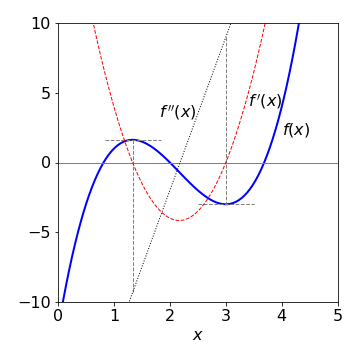Figure 12. The curve $$f(x) = 2(x - 2)^3 - x^2 + 4$$ showing its maximum and minimum, and its first $$f'(x)$$ and second $$f''(x)$$ derivatives. Notice how the first derivative is zero at the maximum and also the minimum and that the second derivative determines which is which in cases when a plot is not made.

## 7.1 Summary#

A function $$f(x)$$ has a maximum or minimum when $$\displaystyle \frac{d}{dx}f(x)=0 \qquad\tag{25}$$

The maximum occurs when $$\displaystyle \frac{d^2}{dx^2}f(x) \lt 0\qquad\tag{26}$$

The minimum occurs when $$\displaystyle \frac{d^2}{dx^2}f(x) \gt 0 \qquad\tag{27}$$

If both first and second derivatives are zero at the same $$x$$ then this is a point of inflexion.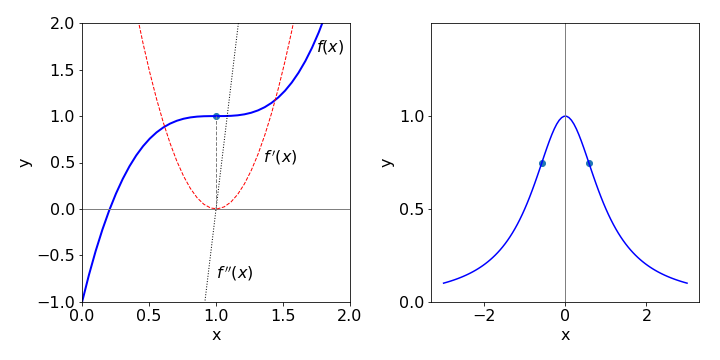Figure. 13(a) Left. A point of inflexion occurs when both first and second derivatives are 0 at the same point on the curve, in this curve $$y=2(x-1)^3$$ +1 this is at the point {1,1}. Fig 13b Right. shows the curve $$y=1/(1+x^2)$$ and its inflexion points.

The curve $$\displaystyle y=\frac{1}{1+x^2}$$ has inflection points as shown in fig 13(b). The first and second derivatives are

$\displaystyle y'=-\frac{2x}{(1+x^2)^2}, \qquad y''=\frac{6x^2-2}{(1+x^2)^3}$

The first derivative is zero at $$x=0$$, and the second zero at $$\displaystyle x=\pm\frac{1}{\sqrt{3}}$$ and $$\displaystyle y=\pm\frac{3}{4}$$

### Luminescence from phosphors. An example with differentiation of an integral and numerically solving for a root#

Many non metallic compounds such as ZnS-Cu and zinc silicates-Mn, will show phosphorescence lasting many tens of seconds after excitation with UV light. Some phosphors also show luminescence on heating and this provides a method of dating minerals and clays directly or in man-made objects discovered by archaeologists. The cause of the luminescence is the recombination of electrons, liberated by the UV or heat, with relatively low energy impurity ‘traps’ and the excess energy is released as a photon. These impurity traps have an energy between the filled levels (ground state or valance band) and the empty ones at higher energy (excited state or conduction band).

If there are $$n$$ electrons liberated their decay into a trap can be described by a first order scheme as,

$\displaystyle \frac{dn}{dt}=-k_1n$

where $$k_1$$ is the rate constant. This itself is given by an Arrhenius expression $$k_1=k_0e^{-E/{k_BT}}$$ where $$E$$ is the trap depth giving

$\displaystyle \frac{dn}{dt}=-k_0e^{-E/{k_BT}}n\quad\text{or}\quad \frac{dn}{n}=-k_0e^{-E/{k_BT}}dt\qquad\tag{27a}$

Integrating produces

$\displaystyle n=n_0e^{-k_1t}\quad \text{or} \quad n=n_0 e^{\large -k_0te^{-E/k_BT}} \qquad\tag{27b}$

with $$n_0$$ as the initial number of $$n$$. The intensity of luminescence (photons/sec) is the rate of being trapped, $$-dn/dt$$, or

$\displaystyle k_1n =n_0k_0e^{-E/k_BT} e^{\large -k_0te^{-E/k_BT}}$

Integrating over all time ($$t=0\to \infty$$) produces a total intensity of $$n_0$$, i.e. every $$n$$ has found a trap as is to be expected.

In many experiments the temperature is increased as the luminescence is decaying and the total luminescence measured vs. temperature. The temperature is ramped so that $$dT =\beta dt$$ where $$\beta$$ is the rate of heating, in $$\mathrm{degrees\,s^{-1}}$$.

Integrating the left-hand side of 27a and substituting for $$dt$$ into the right hand side and integrating gives,

$\displaystyle \ln\left(\frac{n}{n_0}\right)=-\frac{k_0}{\beta}\int_0^Te^{-E/k_BT}dT,\qquad n=n_0\exp\left(-\frac{k_0}{\beta}\int_0^Te^{-E/k_BT}dT\right)$

The luminescence intensity is proportional to the rate of supply of electrons to the traps and is thus proportional to $$-dn/dt$$ making the intensity at temperature $$T$$

$\displaystyle I_T = n_0k_0e^{-E/k_BT} e^{-(k_0/\beta){\large\int_0^Te^{-E/k_BT}}dT}\qquad\tag{27c}$

The intensity $$I_T$$ has units of photons/sec. If this is divided by the heating rate $$\beta$$ i.e. $$I_T/\beta$$ is then the number of photons liberated per degree and when integrated over all temperatures produces $$n_0$$ the total number of electrons present initially. Fig 13b shows plots of $$I_T/\beta$$ vs. temperature.

The intensity must always be positive, because negative intensity is not physically realistic, and also has a maximum. We can understand this directly without calculation. At low temperatures no electrons have the energy to surmount the energy barrier making the luminescence zero. At very high temperatures all the electrons have enough energy to reach a trap and no more are left to luminesce and so again the intensity is zero. This is important when this method is used to date pottery or other artefacts because heating sets the ‘clock back to zero’ by allowing all electrons to recombine with traps. Thermally and/or by exposure to light the electrons are released and so the material can luminesce again. This provides a way of dating.

Mathematically, when $$T\to\infty$$ in the limit the integral in $$T$$ becomes $$\infty$$ and thus the exponential becomes $$e^{-\infty}=0$$ and the luminescence intensity is zero. When $$T\to 0$$ the first exponential becomes zero, $$e^{-E/0}=e^{-\infty}=0$$ and $$I_T=0$$.

To find the temperature of maximum luminescence the equation for the intensity is differentiated and set to zero. This will use some of the methods described so far, product rule and differentiating an integral (see 3.15), viz. $$\displaystyle \frac{d}{dx}\int_a^x f(u)du =f(x)$$ and so,

$\displaystyle \frac{dI_T}{dT}=n_0k_0\frac{E}{k_BT^2}e^{-E/k_BT}\left( e^{-{\large\int_0^T\frac{k_0}{\beta}e^{-E/k_BT}}dT}\right)-n_0k_0e^{-E/k_BT}\left( e^{-{\large\int_0^T\frac{k_0}{\beta}e^{-E/k_BT}}dT} \right)\frac{k_0}{\beta}e^{-E/k_BT} =0$

which greatly simplifies to

$\displaystyle k_0k_BT_m^2e^{-E/(k_BT_m)}-E\beta=0$

This result can only be solved numerically, such as by the Newton-Raphson method, to find the trap depth, $$E$$ when the maximum temperature $$T_m$$ is known from experiment.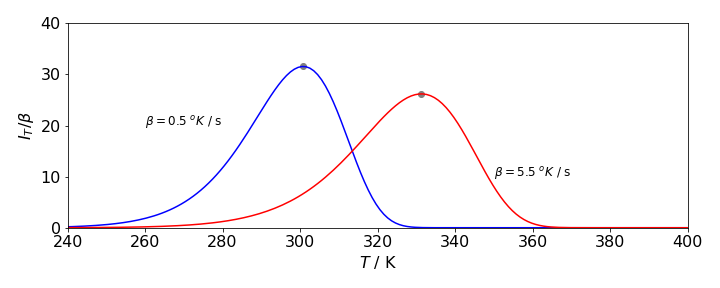Figure 13b. Luminescence heating curves from eqn 27c for a crystal containing impurity traps of depth $$E$$. The intensity divided by the rate of heating is plotted. This means that the curves show the number of photons liberated at each temperature and so the area under each curve is $$n_0$$ the total number of electrons present initially. Recombination of electrons and traps produces a photon. The parameters user were $$n_0=1000,\; k_0 = 3\cdot 10^9\,\mathrm{s^{-1}}$$, $$E = 5000\,\mathrm{cm^{-1}}$$ the heating rate $$\beta=0.5$$ and $$5.5$$ degrees/second is shown on the plot. The temperature at the maximun luminescence intensity is $$300.8$$ and $$331.3$$ K respectively for the $$\beta$$ shown and were calculated using the Newton-Raphson method.

## 8 The Calculus of Variations#

Instead of finding the maximum or minimum of a curve, consider finding the shortest distance between two points on the earth’s surface or on a cone, or finding the equation giving the minimum area of a surface, or the curve of fastest descent between two points. The Calculus of Variations allows us to work out solutions to problems of this type.

You can imagine a graph that shows the many possible different curves that will fit between any two points. A practical example would be all the different routes by which you could travel from, say, Paris to Berlin. The calculus of variations is so named because it varies any path by an amount $$\delta y$$ and, as the variation, $$\delta y \to 0$$, the path along $$y$$ becomes the same as that along $$y + \delta y$$. Because the whole path is sought, the problem is to find a function $$f$$ that, provided that it exists, will make an integral have a stationary value, also called an extremal, which usually means that it has the smallest possible value. This condition is written as

$\displaystyle I=\int_a^b f\left(x,y,\frac{dy}{dx}\right)\; dx \qquad\tag{28}$

Notice that the function $$f(\cdots)$$ normally includes three terms, one in the independent variable $$x$$, the next in the dependent one $$y$$ and the last in the derivative $$dy/dx$$. Although this equation contains an integral, when solved to find its minimum, differentiation is mainly involved. You may need to consult Chapter 4 on integration to complete the last step in this type of problem.

Consider, for example, finding the equation that describes the minimum value of all possible surfaces of revolution. A surface of revolution is the surface obtained by rotating a curve, such as a parabola, about an axis; Fig. 8 (Q31) shows the shape of a parabola and its surface of revolution is shaped somewhat like a bowl. Whatever the equation, $$y = \cdots$$ is, the surface area is always given by the integral

$\displaystyle 2\pi \int_a^b y ds=2\pi \int_a^b y\sqrt{1+\left( \frac{dy}{dx} \right)^2}dx \qquad\tag{29}$

and a straightforward integration with the parabola $$y = 2\sqrt{ax}$$ will produce the parabola’s surface area. The term in the square root is the length of a small element of the curve, see Fig. 19 (Q51) and the integral has the form of equation (28).

Imagine now a surface film suspended between two similar wire hoops at $$x = a$$ and $$b$$, in practice this could be a soap film, see Fig. 13(b). Now, suppose that the problem is to find that one particular curve, of all possible curves, that will produce the minimum surface area within the two rings; this minimum area surface is the surface formed by a soap film, and its profile is called the Catenary. The equation for the film $$y = \cdots$$ was not known before starting the calculation. The calculus of variations allows it be found by first finding $$dy/dx$$ and then integrating it.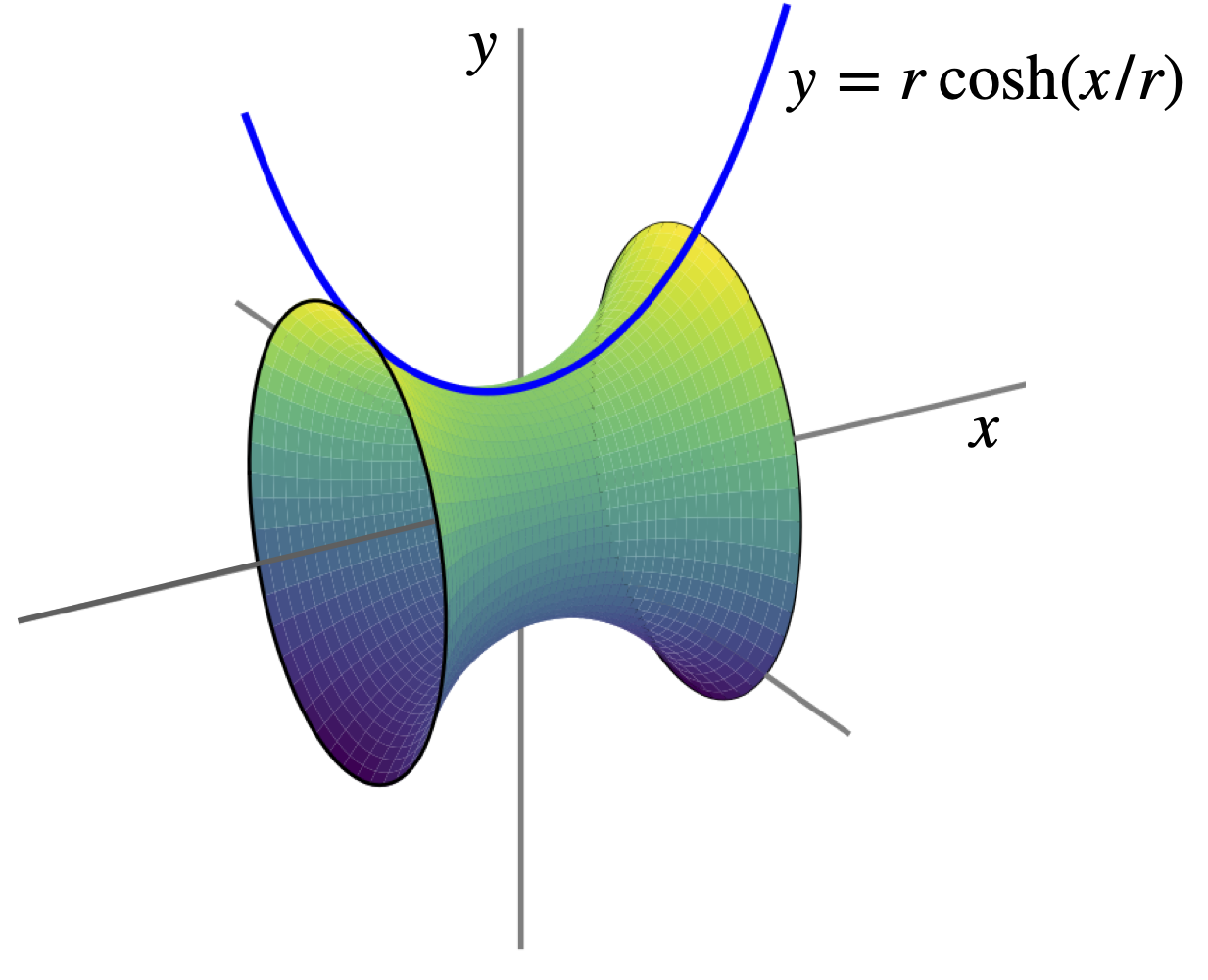Figure 13(b). Surface of revolution, soap film between rings of radius $$r$$.

The calculus of variations defines a formula, variously called the Euler or the Euler - Lagrange equation, by which it is possible to evaluate the integral (28) so that it has its minimum value. The Euler equation is

$\displaystyle \frac{\partial f}{\partial y}-\frac{d}{dx}\frac{\partial f}{\partial y_x}=0 \qquad\tag{30}$

where in this instance the function $$f$$ is

$\displaystyle f= y\sqrt{1+\left( \frac{dy}{dx} \right)^2}$

and $$y_x = dy/dx$$ is the expression we want to find, which, when integrated, produces $$y$$, the equation of the curve required. Notice that the equation tells us to differentiate with respect to $$dy_x$$; see Section 5.6, which describes differentiation with respect to a function. Should the equation for $$f$$ not explicitly contain $$x$$ then a simpler version can be used which is

$\displaystyle \frac{\partial f}{\partial y}-\frac{d}{dx}\left(f-y_x\frac{\partial f}{\partial y_x}\right)=0 \qquad\tag{31}$

and the extremal is found from

$\displaystyle f-y_x\frac{\partial f}{\partial y_x} = const \qquad\tag{32}$

This is sometimes called the ‘Beltrami’ identity. The words ‘not explicit’ mean that $$f$$ is a function of $$y$$ or $$dy/dx$$ such as $$f = y^2 + dy/dx$$ which does not explicitly depend on $$x$$; the function $$f = x^2$$ explicitly depends on $$x$$.

## 8.1 Calculating the shape of the minimum surface of revolution.#

Using the Euler equation is actually not that difficult and as an example the minimum surface of revolution of all possible surfaces for a film suspended between two rings is calculated. A surface of revolution is given by equation (29) but if the surface is to be a minimum then (30) or (32) must also apply. The latter equation is easier to use with $$f =y \sqrt{1 + (dy/dx)^2}$$ because this does not explicitly contain $$x$$.

Using the notation $$\displaystyle y_x = \frac{dy}{dx}$$ then $$f =y \sqrt{1 + y_x^2}$$ and the derivative in eqn 32 becomes,

$\displaystyle \frac{\partial f }{\partial y_x} = \frac{1}{2}(1+y_x^2)^{-1/2}2y_x$

Substituting into eqn 32 and changing to full notation produces

$\displaystyle y\left[ 1+\left(\frac{dy}{dx}\right)^2 \right]^{1/2} - y\left(\frac{dy}{dx} \right)^2 \left[ 1+\left(\frac{dy}{dx}\right)^2 \right]^{-1/2}=y\left[ 1+\left(\frac{dy}{dx} \right)^2\right]^{-1/2}= a$

where $$a$$ is a constant. Rearranging the result leads to

$\displaystyle \frac{dy}{dx}= \sqrt{ \left( \frac{y}{a} \right)^2 - 1 }$

which, when integrated, gives

$\displaystyle y = a \cosh\left(\frac{x}{a} + b\right)$

where $$b$$ is a constant of integration. The constant $$b$$ sets the position of the minimum of the curve; $$b = 0$$ sets the minimum at $$x = 0$$, whereas $$a$$ determines the depth of the curve . This equation describes the Catenary or the curve describing the shape of the edge of the minimum surface of revolution as in a soap film supported on rings, see fig 13(b). It is also the shape produced by a flexible cable or chain hanging under its own weight i.e. under a constant uniform force such as gravity. The shape is very close to, but distinct from that of parabola.

## 8.2 The Brachistochrone, the Tautochrone and the Cycloid#

The Brachistochrone is the name of the curve a frictionless particle will travel along to pass between two points in the shortest time when acted on by a force such as gravity. The time taken is much less than that taken to move down a straight slope or, in fact, any other slope. The Tautochrone is the same curve but is used to describe the fact that a particle set in motion down the curve will arrive at the horizontal part at the same time irrespective of where it starts from. The inverted Brachistochrone is the cycloid, see question 53.

As it will simplify the calculation, we will suppose that a particle of mass $$m$$ travels downwards in the +$$x$$ direction and moves to the right as the +$$y$$ direction after starting at rest at the origin (Margenau & Murphy, 1943). Using conservation of potential and kinetic energy,

$\displaystyle mgx = \frac{mv^2}{2}$

where $$v$$ is the velocity at any point on the path and $$g$$ the acceleration due to gravity. The velocity is found starting with a small (infinitesimal) element $$ds$$ of the path and using Pythagoras ($$\displaystyle ds^2=dx^2+dy^2$$) is therefore valid and gives

$\displaystyle v=\frac{ds}{dt}=\frac{\sqrt{dx^2+dy^2}}{dt}$

then substituting for $$v$$ and rearranging gives the change in time,

$\displaystyle dt= \frac{\sqrt{1+(dy/dx)^2} }{\sqrt{2gx}}dx$

The integral to minimize is therefore the time producing

$\displaystyle t=\frac{1}{\sqrt{2g}}\int_{x_0}^{x} \frac{\sqrt{1+y_x^2}}{\sqrt{x}}dx$

where $$y_x \equiv dy/dx$$. The constant $$2g$$ can be ignored for it will not enter into the shape of the curve. The result of the calculation is an equation $$y$$ vs $$x$$ that the particle follows.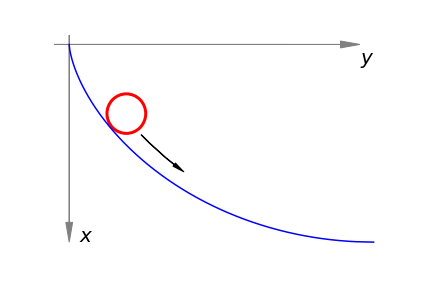Figure 14. Part of the Brachistochrone, notice that the axes are rotated clockwise by $$90$$ degrees compared to convention.

Using the Euler equation (30) with $$\displaystyle f=\frac{\sqrt{1+y_x^2}}{\sqrt{x}}$$ and because $$\displaystyle df/dy$$=0, this leaves just the second term in eqn. 30 as

$\displaystyle \frac{d}{dx}\left(\frac{y_x}{\sqrt{x}\sqrt{1+y_x^2}} \right) =0$

To work towards obtaining the equation for $$y$$ this equation is integrated once to produce

$\displaystyle \frac{y_x}{\sqrt{x}\sqrt{1+y_x^2}}=\sqrt{c}$

The constant of integration is chosen as $$\sqrt{c}$$ rather than $$c$$ to make the following equations simpler. To find $$y_x=dy/dx$$ both sides are squared and after multiplying top and bottom inside the square root by $$(1-cx)$$ and rearranging this gives

$\displaystyle \frac{dy}{dx}={\frac{\sqrt{cx(1-cx)}}{1-cx}}$

Integrating produces with $$cx\lt 1$$,

$\displaystyle y=\frac{\sin^{-1}\left(\sqrt{1-cx}\right)}{c}+\sqrt{\frac{x(1-cx)}{c}}+c_1$

where $$c_1$$ is a second constant of integration. If the particle starts at the origin then $$y = 0$$ when $$x = 0$$ and therefore $$c_1 = -\pi/2c$$. This is the curve shown in fig. 14 but plotted with axes as shown and not in the conventional way.

The solution to this problem was found independently by the Bernoulli brothers, and by Leibniz, Huygens and Newton towards the end of the seventeenth and beginning of the eighteenth centuries.